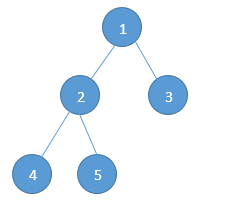Home Trees Given a binary tree, find the sum of all its elements without using recursion.

# Given a binary tree, find the sum of all its elements without using recursion.

Question: Given the root pointer to a binary tree, find the sum of all its elements without using recursion.

Input: Sample Tree (Pointer to node 1 is given).Output: Sum = 15

We discussed how to find the sum of all elements of a binary tree using recursion in this post.

If recursion seems tough to you , you can always go by the iterative approach and use Level Order Traversal of binary tree. While performing a level order traversal, we just need to create a variable that stores the sum of all elements. Upon removing each element from the queue, just add it to the sum. Here is an implementation of the method described above.

int sumOfBinaryTree(struct binaryTreeNode * root)
{
// Level order traversal
struct binaryTreeNode * temp = NULL;
struct queue * Q = NULL;

// Maintain a sum
int sum = 0;

if(root == NULL)
return 0;

Q = enQueue(Q, root);
while(!isQueueEmpty(Q))
{
temp = Q -> front -> data;

// Add it to the sum
sum += temp -> data;

Q = deQueue(Q);
if(temp -> left)
Q = enQueue(Q, temp -> left);
if(temp -> right)
Q = enQueue(Q, temp -> right);
}
// Delete the queue
free(Q);

// Now return the count
return sum;
}


Time Complexity:- O(n)
Space Complexity:- O(n)

Ideone link for the running code:- http://ideone.com/IfbxoG

#### You may also likeMarch 16, 2015 - 09:04

I believe there is a mistake at line 16 of the code ,it should be temp->data instead of tempMarch 16, 2015 - 16:38

Hi Shantun,

The code is completely correct. The running link has also been provided for help. :)